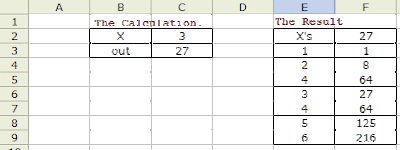### Excel Function :TABLE

Its been a while since I have done some posting. This option in excel is something which I thought would be very useful for many.

Its the TABLE Function in MS Excel. This is especially useful in the following scenarios.

Assume you have a very lengthy calculation to arrive at a value. And you want to see how one (or two) input variables affect the output. One way to do this is to have each calculation in adjacent columns and populate the rows with varying input value. Also, if you want two inputs to be changed, its even more difficult. Using TABLE, you can do this very easily.

I even remember that I had written a whole set of VBA codes to manage what TABLE could do!

Enough of introduction....

(A) How to do a 1D TABLE (one variable is changed).Step 1: Basic Calculation

Setup your basic calculation with the variable value in separate cell(s).
In the Eg, Input is in [C2] and output is in [C3]

Step 2: Prepare the Table

Prepare the frame for the Table. Different possible values of the variable is arranged in a column ( assume [E3:E10] ). Immediate to the right of these values ( [F3:F10] ) will be the place for the results. Link the result cell of Step1 to the cell just above it [F2] (for eg, =C3).

Step 3: Populate the Table

Select the full table. ( [E2:F3] ).
Go to Data -> Table.
Select the input variable cell as Column Input Cell ([C2])
Select OK.

You are DONE.

(B) How to do a 2D TABLE (two variables are varied).Step 1: Stays the same.

Step 2: Populate the possibilities of variable 1 in the 1st column and variable 2 as 1st row. The output cell should be linked to the Top-Left Corner.

Step 3: Select the two variable reference cells in the dialog. Click OK and you should be DONE.

If there is some mismatch, please repeat the step 3 with the reference cells interchanged.

Hope its useful.. Any questions?, let me know...Anonymous said…
Thanx alot. It helped me.Anonymous said…
Many thanks. Very useful!Anonymous said…
Thank you..... but how it works when is complex?? example when you have a matrix and you need to bring a value that depens on another variables and you just need a brief of the big one?
A specific example, you have a financial model structured and you need to have a simply table with the information of NPV and IRR as a resume of whole with the possibility to change the % and get the new resultls.... How can I make that Table function works?Joe said…
Hi Anonymous !!!
I cant figure out the question. Please give an example and I can try.

Joe

### Proe Function / Relation to round the decimal places

Its strange that ProE does not have a ROUND function. At least I counldnt find it. It has two funtions. Ceil will always round it up. Floor will always round it down. I have used both to get the ROUND functionality. here is the relation...

### TreeView Structure in Excel VBA

TreeView is extreamly useful in specific cases but it can be bit tricky sometimes to implement.  Last Few days I was working on a TreeView Structure and thought I will share the knowledge I gained...  This post takes you through the basic operations to create and operate a TreeView. It will be like the Folder tree window of the windows explorer.  Moreover, you can have it dynamically updated based on the data in excel.... Here we go..